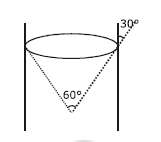# A capillary tube made of glass of radius 0.15 mm is dipped vertically in a beaker filled with methylene iodide (surface tension = 0.05 Nm–1, density = 667 kg m–3) which rises to height h in the tube. It is observed that the two tangents drawn from liquid-glass interfaces (from opp. sides of the capillary) make an angle of 60° with one another. Then h is close to (g=10 ms–2).

1) 0.172 m

2) 0.049 m

3) 0.087 m

4) 0.137 m$$h=\frac{2Tcos\theta }{\rho gr}\left \{ \theta =30^{0} \right \}$$ $$h=\frac{2\times 0.05\times \times \frac{\sqrt{3}}{2} }{667\times 10\times 0.15\times 10^{-3}}$$

=0.087 m(0)(0)